# An Introduction to Bonds, Bond Valuation & Bond Pricing

Bonds form a significant portion of the financial market and are a key source of capital for the corporate world. Therefore every corporate finance course in the MBA program will introduce students to bonds at varying depth. We have provided a quick outline of what a student will need to know to understand bonds and the pricing or valuation of bonds which is the primary focus in the initial corporate finance program. More advanced finance courses will introduce students to advanced bond concepts including duration, managing bond portfolios, understanding and interpreting term structures, etc.

## 1) What are Bonds?

A bond is a debt instrument that provides a periodic stream of interest payments to investors while repaying the principal sum on a specified maturity date.  A bond’s terms and conditions are contained in a legal contract between the buyer and the seller, known as the indenture.

## 2) Key Bond Characteristics

Each bond can be characterized by several factors.  These include:

• Face Value
• Coupon Rate
• Coupon
• Maturity
• Call Provisions
• Put Provisions
• Sinking Fund Provisions

a) Face Value

The face value (also known as the par value) of a bond is the price at which the bond is sold to investors when first issued; it is also the price at which the bond is redeemed at maturity.  In the U.S., the face value is usually \$1,000 or a multiple of \$1,000.

b) Coupon Rate

The periodic interest payments promised to bond holders are computed as a fixed percentage of the bond’s face value; this percentage is known as the coupon rate.

c) Coupon

A bond’s coupon is the dollar value of the periodic interest payment promised to bondholders; this equals the coupon rate times the face value of the bond. For example, if a bond issuer promises to pay an annual coupon rate of 5% to bond holders and the face value of the bond is \$1,000, the bond holders are being promised a coupon payment of (0.05)(\$1,000) = \$50 per year.

d) Maturity

A bond’s maturity is the length of time until the principal is scheduled to be repaid.  In the U.S., a bond’s maturity usually does not exceed 30 years.  Occasionally a bond is issued with a much longer maturity; for example, the Walt Disney Company issued a 100-year bond in 1993.  There have also been a few instances of bonds with an infinite maturity; these bonds are known as consols.  With a consol, interest is paid forever, but the principal is never repaid.

e) Call Provisions

Many bonds contain a provision that enables the issuer to buy the bond back from the bondholder at a pre-specified price prior to maturity. This price is known as the call price.  A bond containing a call provision is said to be callable. This provision enables issuers to reduce their interest costs if rates fall after a bond is issued, since existing bonds can then be replaced with lower yielding bonds.  Since a call provision is disadvantageous to the bond holder, the bond will offer a higher yield than an otherwise identical bond with no call provision.

A call provision is known as an embedded option, since it can’t be bought or sold separately from the bond.

f) Put Provisions

Some bonds contain a provision that enables the buyer to sell the bond back to the issuer at a pre-specified price prior to maturity. This price is known as the put price.  A bond containing such a provision is said to be putable.  This provision enables bond holders to benefit from rising interest rates since the bond can be sold and the proceeds reinvested at a higher yield than the original bond.  Since a put provision is advantageous to the bond holder, the bond will offer a lower yield than an otherwise identical bond with no put provision.

g) Sinking Fund Provisions

Some bonds are issued with a provision that requires the issuer to repurchase a fixed percentage of the outstanding bonds each year, regardless of the level of interest rates. A sinking fund reduces the possibility of default; default occurs when a bond issuer is unable to make promised payments in a timely manner.  Since a sinking fund reduces credit risk to bond holders, these bonds can be offered with a lower yield than an otherwise identical bond with no sinking fund.

## 3) Bond Issuers

Bonds are issued by borrowers to raise funds for long-term investments; the main issuers of bonds in the U.S. are:

• The U.S. Treasury
• Corporations
• Municipalities
• Foreign Entities

a) The U.S. Treasury

Treasury securities are issued by the U.S. government to finance its deficits.  These are free of default risk, which is the risk that the investor will not receive all promised payments.  They are not taxed by state and local governments, but are taxed at the federal level.

U.S. Treasuries can be classified by their maturities as follows:

• Treasury bills – the maturity is one year or less; the currently available maturities are 4 weeks, 13 weeks, 26 weeks and 52 weeks
• Treasury notes – the maturity ranges between 1 and 10 years; the currently available maturities are 2, 3, 5, 7 and 10 years
• Treasury bonds – the maturity ranges between 20 and 30 years; the currently available maturity is 30 years

Another key difference between these securities is that Treasury bills are sold at a discount from their face value and redeemed at face value; Treasury notes and bonds are sold and redeemed at face value and pay semi-annual coupons to investors.

b) Corporations

Corporations can raise funds by issuing debt in the form of corporate bonds.  These bonds offer a higher promised coupon rate than Treasuries, but expose investors to default risk.

Ratings agencies, such as Standard and Poor’s and Moody’s, rank corporate issuers according to their likelihood of default.  The riskiest corporations offer the highest coupon rates to investors as compensation for default risk.

c) Municipalities

A municipal bond is issued by a state or local government; as a result, they carry little or no default risk. Occasionally, municipalities do default on their debts; in 2013, the city of Detroit filed for bankruptcy as a result of being unable to pay its debts.

Municipal bonds offer an extremely favorable tax treatment to investors.  They are not taxed by federal, state or local governments as long as the bond holder lives in the municipality in which the bonds were issued.  As a result, municipal bonds can be issued with very low yields.

d) Foreign Entities

Foreign bonds are issued by foreign governments and corporations and are denominated in dollars.  (If they are denominated in a foreign currency, they are known as eurobonds.)  Dollar-denominated bonds issued in the U.S. by foreign entities are known as Yankee Bonds.

## 4) Pricing Bonds

A bond’s price equals the present value of its expected future cash flows. The rate of interest used to discount the bond’s cash flows is known as the yield to maturity (YTM.)

a) Pricing Coupon Bonds

A coupon-bearing bond may be priced with the following formula: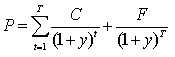where:

C = the periodic coupon payment

y = the yield to maturity (YTM)

F = the bond’s par or face value

t = time

T = the number of periods until the bond’s maturity date

This formula shows that the price of a bond is the present value of its promised cash flows.  As an example, suppose that a bond has a face value of \$1,000, a coupon rate of 4% and a maturity of four years.  The bond makes annual coupon payments.  If the yield to maturity is 4%, the bond’s price is determined as follows: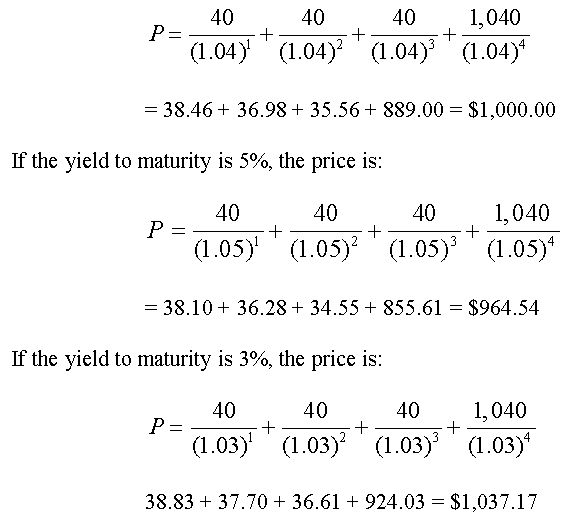These results show the following important relationship:

• if y > coupon rate, P < face value
• if y = coupon rate, P = face value
• if y < coupon rate, P > face value

These results also demonstrate that there is an inverse relationship between yields and bond prices:

• when yields rise, bond prices fall
• when yields fall, bond prices rise

b) Adjusting for Semi-Annual Coupons

For a bond that makes semi-annual coupon payments, the following adjustments must be made to the pricing formula:

• the coupon payment is cut in half
• the yield is cut in half
• the number of periods is doubled

As an example, suppose that a bond has a face value of \$1,000, a coupon rate of 8% and a maturity of two years.  The bond makes semi-annual coupon payments, and the yield to maturity is 6%.  The semi-annual coupon is \$40, the semi-annual yield is 3%, and the number of semi-annual periods is four.  The bond’s price is determined as follows: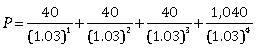= 38.83 + 37.70 + 36.61 + 924.03 = \$1,037.17

As an alternative to this pricing formula, a bond may be priced by treating the coupons as an annuity; the price is therefore equal to the present value of an annuity (the coupons) plus the present value of a sum (the face value.) This method of valuing bonds will use the formula: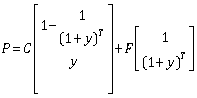The bond in the previous example can be priced using this alternate bond valuation formula as follows: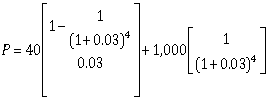= 148.68 + 888.49 = \$1,037.17

c) Pricing Zero Coupon Bonds

A zero-coupon bond does not make any coupon payments; instead, it is sold to investors at a discount from face value. The difference between the price paid for the bond and the face value, known as a capital gain, is the return to the investor. The pricing formula for a zero coupon bond is: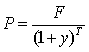As an example, suppose that a one-year zero-coupon bond is issued with a face value of \$1,000.  The discount rate for this bond is 8%.  What is the market price of this bond?  In order to be consistent with coupon-bearing bonds, where coupons are typically made on a semi-annual basis, the yield will be divided by 2, and the number of periods will be multiplied by 2: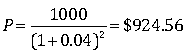## 5) Yield Measures

There are different types of yield measures that may be used to represent the approximate return to a bond.  These include:

• yield to maturity (YTM)
• yield to call (YTC)
• current yield

a) Yield to Maturity (YTM)

The discount rate used in the bond pricing formula is also known as the bond’s yield to maturity (YTM) or yield.  This equals the rate of return earned by a bond holder (known as the holding period return) if:

• the bond is held to maturity
• the coupon payments are reinvested at the yield to maturity

A bond’s YTM is the unique discount rate at which the market price of the bond equals the present value of the bond’s cash flows:

Market Price = PV (Cash Flows)

The yield to maturity of a bond can be determined from the bond’s market price, maturity, coupon rate and face value.  As an example, suppose that a bond has a face value of \$1,000 and will mature in ten years.  The annual coupon rate is 5%; the bond makes semi-annual coupon payments.  With a price of \$950, what is the bond’s yield to maturity?

It is impossible to solve for the yield to maturity algebraically; instead, this must be done using a financial calculator or Microsoft Excel.  For example, a bond’s yield to maturity can be computed in Excel using the RATE function:

= RATE(nper, pmt, pv, [fv], [type], [guess])

where:

nper = number of periods

pmt = periodic payment

pv = present value

fv = future value

type = 0 for ordinary annuity

1 for annuity due

guess = initial guess

The variables in brackets (fv, type and guess) are optional values; the value of type is set to zero if it is not specified.  Guess can be used to provide an initial estimate of the rate, which could potentially speed up the calculation time.

Note that either pv or fv must be negative, and the other must be positive.  The negative value is considered to be a cash outflow, and the positive value is considered to be a cash inflow.

Also note that entering semi-annual periods and coupon payments will produce a semi-annual yield; in order to convert this into an annual yield (on a bond-equivalent basis), the semi-annual yield is doubled.

In this example,

nper = 20

pmt = \$25

pv = \$950

fv = \$1,000

At a price of \$950, the semi-annual yield to maturity is:

=RATE(nper, pmt, pv, [fv], [type], [guess])

= RATE(20, 25, -950, 1000)

= 2.83%

The annual yield is (2.83%)(2) = 5.66%

At a price of \$1000, the semi-annual yield to maturity is:

=RATE(nper, pmt, pv, [fv], [type], [guess])

= RATE(20, 25, -1000, 1000)

= 2.50%

The annual yield is (2.50%)(2) = 5.00%

At a price of \$1050, the semi-annual yield to maturity is:

=RATE(nper, pmt, pv, [fv], [type], [guess])

= RATE(20, 25, -1050, 1000)

= 2.19%

The annual yield is (2.19%)(2) = 4.38%

b) Yield to Call (YTC)

For a bond that is callable, the yield to call may be used as a measure of return instead of the yield to maturity. The process is similar to computing yield to maturity, except that the maturity date of the bond is replaced with the next call date.  This is because yield to call is based on the assumption that the bond will be called on the next call date.  The face value is replaced with the call price since this is the amount that the investor will receive if the bond is called.

As an example, suppose that a ten-year bond was issued two years ago and is callable in three years at a price of \$1,100.  The bond’s face value is \$1,000 and its coupon rate is 7%.  Coupons are paid on an annual basis; the current market price of the bond is \$1,200.  What is the yield to call?

In this case, the bond will mature in eight years, but it can be called in three years.  If the bond is called, the investor will receive a price of \$1,100 instead of the face value of \$1,000.  The yield to call is computed as follows:

The yield to call is:

=RATE(nper, pmt, pv, [fv], [type], [guess])

= RATE(3, 70, -1200, 1100)

= 3.14%

c) Current Yield

The current yield is simpler measure of the rate of return to a bond than the yield to maturity. Current yield is measured as the ratio of the bond’s annual coupon payment to the bond’s market price.

As an example, suppose that a bond was issued with a coupon rate of 8% and a face value of \$1,000.  The bond has a current market price of \$900. The current yield is computed as:

\$80/\$900 = 8.89%

This measure has the benefit of simplicity.  It suffers from the drawback that it does not account for the time value of money.

6) Excel’s Specialized Bond Functions

Excel contains a set of specialized bond functions that can be used to account for several complications that arise in bond pricing, such as day-count conventions.

a) PRICE

The Excel function price is implemented as follows:

= PRICE(settlement, maturity, rate, yld, redemption, frequency, [basis])

where:

settlement = date on which the bond owner pays for the bond

maturity = maturity date of the bond; this is the date on which the owner receives the principal

rate = annual coupon rate; this is the percentage of face value that is paid to the bond owner each year

yld = the bond’s yield to maturity

redemption = face value (as a percentage of 100)

frequency = the number of coupon payments per year; annually = 1, semi-annually = 2

basis = the day-count convention; the options are:

0 = 30/360

1 = actual/actual

2 = actual/360

3 = actual/365

4 = European 30/360

Treasury bonds follow the actual/actual convention.  Corporate and municipal bonds follow the 30/360 convention.  Money market instruments (e.g., Treasury bills, commercial paper, etc.) follow the actual/360 convention.

Note that the settlement date and maturity date are represented as numerical values in Excel.  The date January 1, 1900 is represented as 1; all later dates represent the number of days that have passed since January 1, 1900.

As an example, suppose that a bond is sold on June 15, 2016 with a maturity date of June 15, 2036.  The coupon rate is 8%, the yield is 9%, the face value is \$1,000 and the bond makes semi-annual coupon payments.  Also assume that the bond uses a 30/360 day count convention for computing coupon payments.  What is the price of the bond?

This is computed with the PRICE function as follows:

= PRICE(settlement, maturity, rate, yld, redemption, frequency, [basis])

= PRICE(42536, 49841, 8%, 9%, 100, 2, 0)

= \$907.99

b) YIELD

The Excel function yield is implemented as follows:

= YIELD(settlement, maturity, rate, pr, redemption, frequency, [basis])

where:

pr = price (as a percentage of face value)

As an example, for the bond in the previous example, the yield can be determined as follows:

= YIELD(settlement, maturity, rate, pr, redemption, frequency, [basis])

= YIELD(42536, 49841, 8%, 90.799, 100, 2, 0)

= 9.00%

Note that the price of the bond is entered as 90.799 instead of 907.99; this indicates that the price is 90.799 percent of the face value.  Also note that 42536 represents June 15, 2016 and 49841 represents 49841.  These numerical values can be obtained with the Excel function DATEVALUE.  For example,

= DATEVALUE(“6/15/16”)

= 42536

This article is one part of a series on fixed income portfolios. Other articles in this series include:

We have provided you with a quick introduction to bonds, bond valuation and the concepts used in pricing bonds. If you have questions or need help understanding bonds, bond valuation or how bonds are priced, please feel free to call our corporate finance tutoring team and one of our CFA or MBA tutors will be happy to assist you.